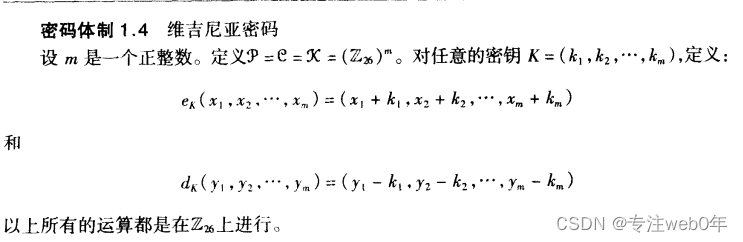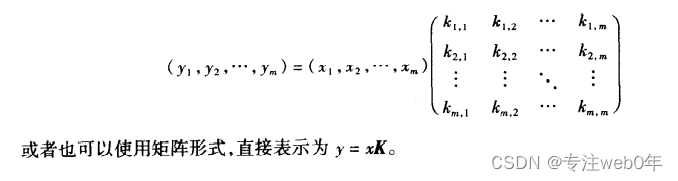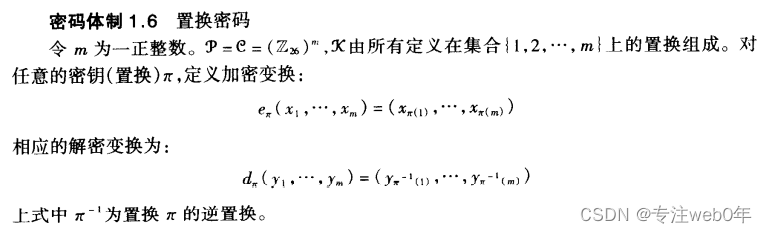# 密码学原理与实践4

## 维吉尼亚密码$$加密是把\mathcal{P}和\mathcal{K}中对应的元素相加，即p_i-k_i$$

## 希尔密码

$$\mathcal{P}=\mathcal{C}=(\mathbb{Z}{26})^m\ 为什么是(\mathbb{Z} {26})^m，因为可以表示多个组合\ 每个组合的每个元素都在一个\mathbb{Z}_{26}中，前面的维吉尼亚密码也是这样的。\ \mathcal{P}、\mathcal{C}、\mathcal{K}，以矩阵形式进行计算，例子如下$$$$x_1\times k_{1,2}中，k_{1,2}的1表示x_1，它的2表示y_2，就是k第一个下标表示加密哪个原文元素，\ 第二个表示得到密文的哪个元素（的一部分，因为要多个加起来才是密文的一个元素)$$

## 置换密码$$置换密码是一种希尔密码，\mathcal{P}、\mathcal{C}、\mathcal{K}都可以看成矩阵，解密就求逆矩阵。$$